徐小夕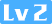817 0 0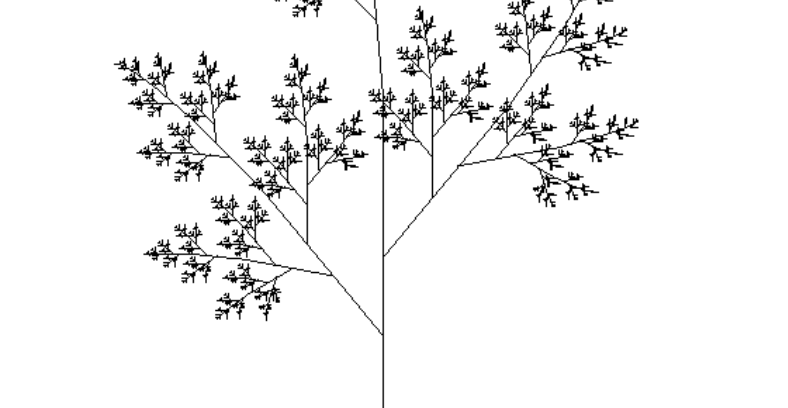### 递归和尾递归

``````function factorial(n) {
if (n === 1) return 1;
return n * factorial(n - 1);
}

factorial(5) // 120``````

``````function factorial(n, total = 1) {
return factorial(n - 1, n * total);
}

factorial(5) // 120``````

### 递归的常用应用案例

#### 1. 数组求和

``````function sumArray(arr, total) {
if(arr.length === 1) {
}
return sum(arr, total + arr.pop())
}

let arr = [1,2,3,4];
sumArray(arr, arr) // 10``````

#### 2. 斐波那且数列

``````// 斐波那契数列
function factorial1 (n) {
if(n <= 2){
return 1
}
return factorial1(n-1) + factorial1(n-2)
}

// 尾递归优化后
function factorial2 (n, start = 1, total = 1) {
if(n <= 2){
}
return factorial2 (n -1, total, total + start)
}``````

#### 5. 深拷贝

`````` function clone(target) {
if (typeof target === 'object') {
let cloneTarget = Array.isArray(target) ? [] : {};
for (const key in target) {
cloneTarget[key] = clone(target[key]);
}
return cloneTarget;
} else {
return target;
}
};``````

#### 6. 爬梯问题

``````n =1; result = 1  --> 1
n =2; result = 2  --> 11 2
n =3; result = 3  --> 111 12 21
...

function steps(n) {
if(n <= 1) {
return 1
}
return steps(n-1) + steps(n-2)
}``````

#### 7. 对象数据格式化

``````let obj = {
a: '1',
b: {
c: '2',
D: {
E: '3'
}
}
}

let obj = {
a: '1',
b: {
c: '2',
d: {
e: '3'
}
}
}

// 代码实现
function keysLower(obj) {
let reg = new RegExp("([A-Z]+)", "g");
for (let key in obj) {
if (obj.hasOwnProperty(key)) {
let temp = obj[key];
if (reg.test(key.toString())) {
// 将修改后的属性名重新赋值给temp，并在对象obj内添加一个转换后的属性
temp = obj[key.replace(reg, function (result) {
return result.toLowerCase()
})] = obj[key];
// 将之前大写的键属性删除
delete obj[key];
}
// 如果属性是对象或者数组，重新执行函数
if (typeof temp === 'object' || Object.prototype.toString.call(temp) === '[object Array]') {
keysLower(temp);
}
}
}
return obj;
};``````

#### 8. 遍历目录/删除目录

``````function deleteFolder(path) {
var files = [];
if(fs.existsSync(path)) { // 如果目录存在
files.forEach(function(file,index){
var curPath = path + "/" + file;
if(fs.statSync(curPath).isDirectory()) { // 如果是目录，则递归
deleteFolder(curPath);
} else { // 删除文件
}
});
fs.rmdirSync(path);
}
}``````

#### 9. 绘制分形图形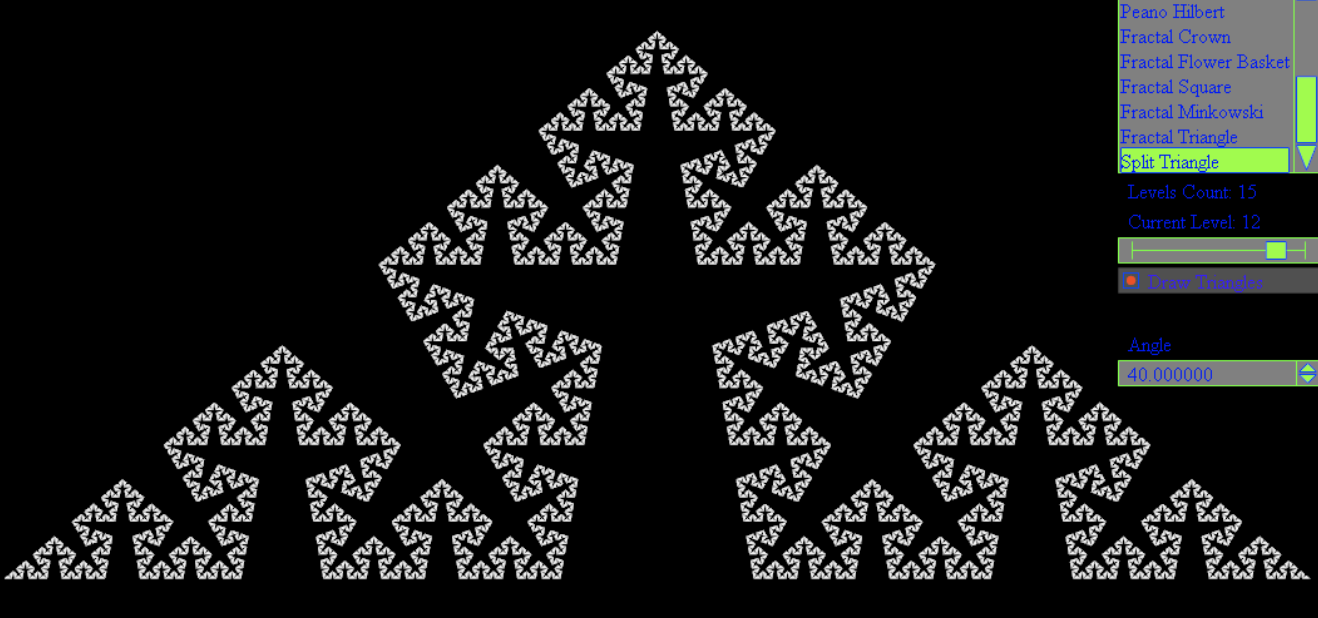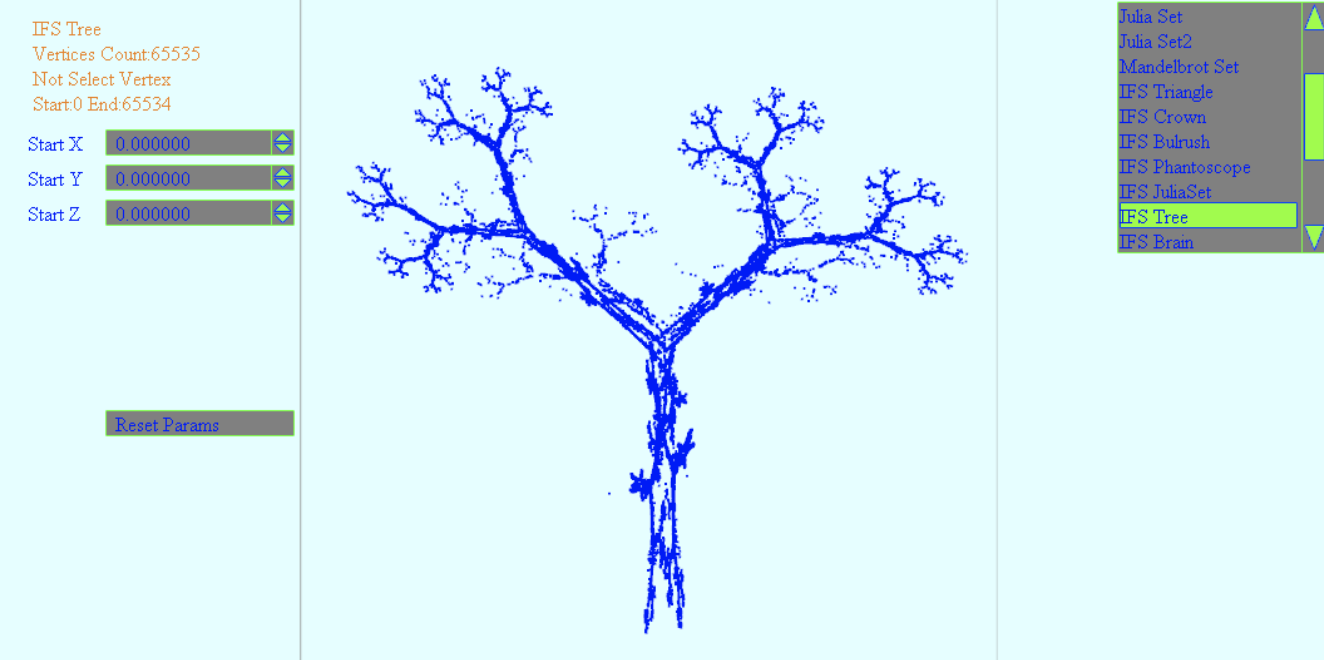我们可以借助一些工具和递归的思想，实现如上的分形图案。

#### 10. 扁平化数组Flat

``````let a = [1,2,3, [1,2,3, [1,2,3]]]
// 变成
let a = [1,2,3,1,2,3,1,2,3]
// 具体实现
function flat(arr = [], result = []) {
arr.forEach(v => {
if(Array.isArray(v)) {
result = result.concat(flat(v, []))
}else {
result.push(v)
}
})
return result
}

flat(a)``````

### 用递归画一棵自定义风格的结构树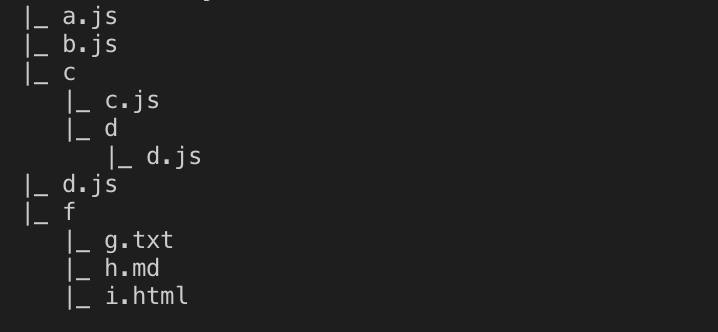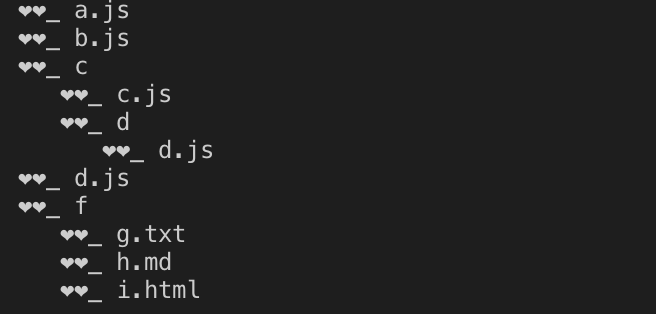该图形是根据目录结构生成的目录树图，在很多应用场景中被广泛使用，接下来我们就来看看他的实现过程吧：

``````const fs = require('fs')
const path = require('path')
// 遍历目录/生成目录树
function treeFolder(path, flag = '|_') {
var files = [];

if(fs.existsSync(path)) {
files.forEach(function(file,index){
var curPath = path + "/" + file;
if(fs.statSync(curPath).isDirectory()) { // recurse
// obj[file] = treeFolder(curPath, {});
console.log(flag, file)
treeFolder(curPath, '   ' + flag)
} else {
// obj['--'] = file
console.log(flag, file)
}
})
// return obj
}
}

treeFolder(path.resolve(__dirname, './test'))``````

test为我们建的测试目录，如下：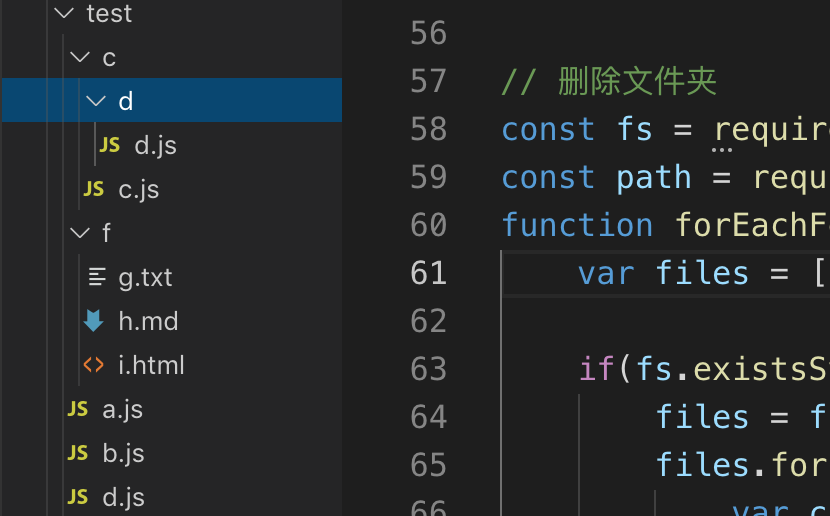我们通过短短10几行代码就实现了一个生成结构树的小应用，是不是感觉递归有点意思呢？在这个函数中，第一个参数是目录的绝对路径，第二个是标示符，标示符决定我们生成的树枝的样式，我们可以自定义不同的样式。

### 相关推荐

4.2 手写Java PriorityQueue 核心源码

《前端实战总结》之使用解释器模式实现获取元素Xpath路径的算法

javascript进阶必备的二叉树知识

Vue - diff 算法
diff是什么？diff就是比较两棵树，render会生成两颗树，一棵新树newVnode，一棵旧树oldVnode，然后两棵树进行对比更新找差异就是diff，全称difference，在vue里面 diff 算法是通过patch函数来完成的，所以有的时候也叫patch算法⏳ diff 发生的时机diff发生在什么时候呢？当然我们可以说在数据更新的时候发生d
JAVA递归实现线索化二叉树
JAVA递归实现线索化二叉树 ============== ### 基础理论 首先，二叉树递归遍历分为先序遍历、中序遍历和后序遍历。 先序遍历为：根节点+左子树+右子树 中序遍历为：左子树+根节点+右子树 后序遍历为：左子树+右子树+根节点 （只要记住根节点在哪里就是什么遍历，且都是先左再右） ### 线索化 现在有这么一棵二叉树，它的数据结
Java乱码
1.Javascript传参乱码： 在浏览器端对要传递的中文参数进行编码处理.代码如下: xmlhttp.open("POST",url,true); //请求参数初始化 xmlhttp.setRequestHeader("Content-Type","application/x-www-form-urlencoded"); //因为请求方式为PO
PTA 是否同一棵二叉搜索树（25 分）
#### 是否同一棵二叉搜索树（25 分） 给定一个插入序列就可以唯一确定一棵二叉搜索树。然而，一棵给定的二叉搜索树却可以由多种不同的插入序列得到。例如分别按照序列{2, 1, 3}和{2, 3, 1}插入初始为空的二叉搜索树，都得到一样的结果。于是对于输入的各种插入序列，你需要判断它们是否能生成一样的二叉搜索树。 ### 输入格式: 输入包含若干组测
PHP数据结构与算法：二叉树
### 一、定义 二叉树是每个节点最多有两个子树的树结构。通常子树被称作“左子树”（left subtree）和“右子树”（right subtree） 。 ### 二、特性 1. 在二叉树的第i层上至多有2^(i-1)个结点（i>0） 2. 深度为k的二叉树至多有2^k - 1个结点（k>0） 3. 对于任意一棵二叉树，如果其叶结点数为N0，而
20155211 网络攻防技术 Exp08 Web基础
20155211 网络攻防技术 Exp08 Web基础 =========================== 实践内容 ---- 1. Web前端HTML，能正常安装、启停Apache。理解HTML，理解表单，理解GET与POST方法,编写一个含有表单的HTML。 2. Web前端javascipt，理解JavaScript的基本功能，理解DOM。
20155211 网络攻防技术 Exp08 Web基础
20155211 网络攻防技术 Exp08 Web基础 =========================== 实践内容 ---- 1. Web前端HTML，能正常安装、启停Apache。理解HTML，理解表单，理解GET与POST方法,编写一个含有表单的HTML。 2. Web前端javascipt，理解JavaScript的基本功能，理解DOM。
LeetCode（110）：平衡二叉树
**Easy！** **题目描述：** 给定一个二叉树，判断它是否是高度平衡的二叉树。 本题中，一棵高度平衡二叉树定义为： > 一个二叉树_每个节点_ 的左右两个子树的高度差的绝对值不超过1。 示例 1: 给定二叉树 `[3,9,20,null,null,15,7]` 3 / \ 9 20 /
React的虚拟DOM

Springmvc异步上传文件
<script src="js/jquery.js" type="text/javascript"></script><script src="js/jquery.ext.js" type="text/javascript"></script><script src="js/jquery.form.js" type="text/javascript"
HelloWorld开发者社区 - 开发者专属的技术社区